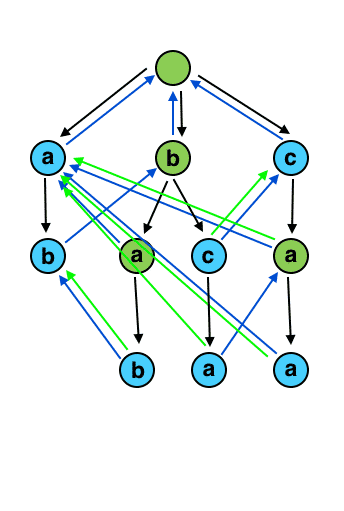## 计蒜客 – 猴子打字

1340 0 2020年3月18日

0 0 投票数

5
jsk
jisuan
suantou
love
program
jisuantouisprogramming

3

AC 自动机算法主要依靠构造一个有限状态机（类似于在一个 Trie 树中添加失配指针）来实现。

#include <bits/stdc++.h>

const int MAXC = 26;
const int MAXN = 1000007;
const int MAX_WORD_LEN = 57;

using namespace std;

// 数据量比较大，可能需要开成全局的，或者动态的，直接放在结构体中，也许会超内存
int child[MAXN][MAXC], fail[MAXN], sta[MAXN], Q[MAXN];
int tot;

/**
* AC 自动机
*/
struct AC_Automaton {

//    int child[MAXN][MAXC], fail[MAXN], sta[MAXN], Q[MAXN];
//    int tot;

/**
* 清空
*/
void clear() {
memset(child, 255, sizeof(child));
memset(fail, 0, sizeof(fail));
tot = 0;
memset(sta, 0, sizeof(sta));
}

/**
* 插入单词
* @param ch 单词，该单词下标从 1 开始
*/
void insert(char* ch) {
int p = 0, l = strlen(ch + 1);
for (int i = 1; i <= l; i++)
{
if (child[p][ch[i] - 'a'] == -1) child[p][ch[i] - 'a']= ++tot;
p = child[p][ch[i] - 'a'];
}
sta[p]++;
}

/**
* 对插入了单词的前缀树构造失败指针
*/
void build() {
int l = 0, r = 0;
for (int i = 0; i < MAXC; i++)
if (child[i] == -1)
child[i] = 0;
else
Q[++ r] = child[i];
while (l < r) {
int p = Q[++l];
for (int i = 0; i < MAXC; i++)
if (child[p][i] == -1)
child[p][i] = child[fail[p]][i];
else {
fail[child[p][i]] = child[fail[p]][i];
Q[++ r] = child[p][i];
}
}
}

/**
* 查询给定的字符串中，一共有多少个单词是出现在词典中的
* @param ch 给定的字符串，该字符串下标从 1 开始
* @return 该字符串中有多少单词是出现在词典中的
*/
int solve(char* ch) {
int ret = 0, p = 0, l = strlen(ch + 1);
for (int i = 1; i <= l; i++) {
p = child[p][ch[i] - 'a'];
int tmp = p;
while (tmp) {
ret += sta[tmp];
sta[tmp] = 0;
tmp = fail[tmp];
}
}
return ret;
}
}T;

int main() {
// 构造 AC 自动机
auto ac = new AC_Automaton();
ac->clear();
int n;
scanf("%d", &n);
// 读入单词，加入前缀树
char* s = (char*)malloc(sizeof(char) * MAX_WORD_LEN);
for (int i = 0; i < n; i++) {
// 字符串下标从 1 开始
scanf("%s", s + 1);
ac->insert(s);
}
// 根据前缀树中的单词构造失败指针，即构造字典
ac->build();
// 给定的文章，下标从 1 开始
char* t = (char*)malloc(sizeof(char) * MAXN);
scanf("%s", t + 1);
printf("%d\n", ac->solve(t));
return 0;
}
0 0 投票数（可选）如果您也有个人网站，不妨分享一下

0 评论

Warning: error_log(/home/wwwroot/www.jxtxzzw.com/wp-content/plugins/spider-analyser/#log/log-1105.txt): failed to open stream: No such file or directory in /home/wwwroot/www.jxtxzzw.com/wp-content/plugins/spider-analyser/spider.class.php on line 2900